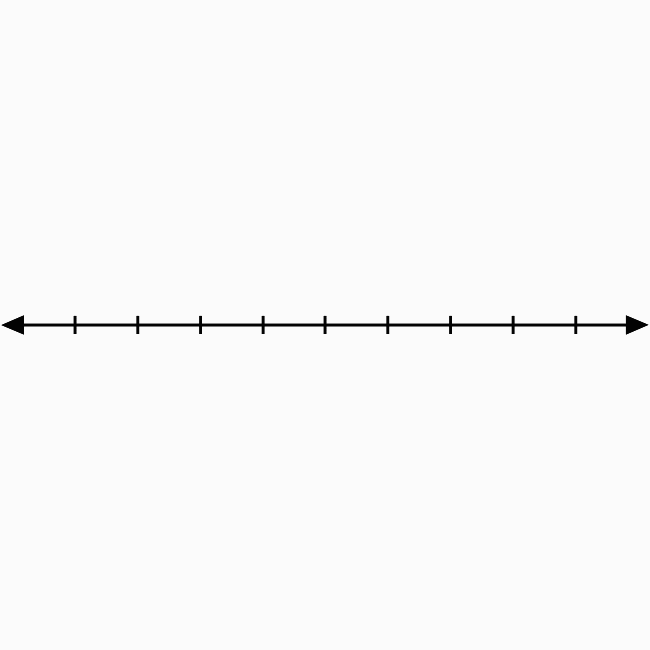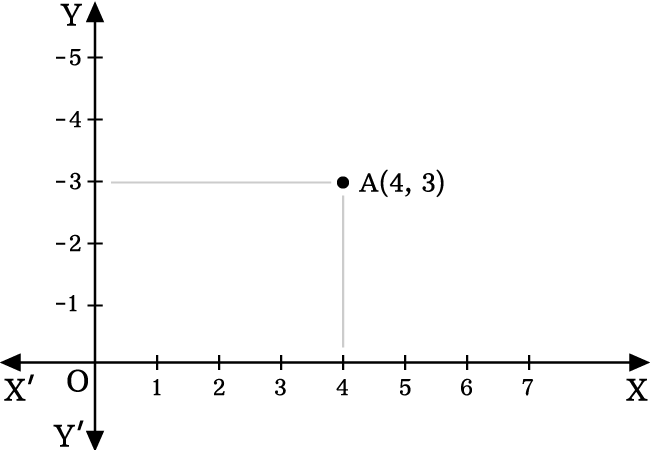The right-top side region in the two dimensional space is called the first quadrant.

## IntroductionTwo number lines are bisected perpendicularly at their centre point in two-dimensional Cartesian coordinate system for splitting the coordinate plane into four equal regions.

The right-top side region is called the first quadrant. The region in the angle $XOY$ is the first quadrant and represented by a Roman numeral $I$.

In $\angle XOY$, the $x$-axis and $y$-axis both represent positive values. Therefore, the signs of abscissa and ordinate of every point in this region must be positive.

If $x$-coordinate and $y$-coordinate of every point are represented by $x$ and $y$ respectively, then the values of them are written as $x > 0$ and $y > 0$ mathematically.

### Usage

The first quadrant is used to identity the location of a point whose abscissa and ordinate are positive. Now, let’s learn how to use the first quadrant in the coordinate geometry.

#### Example

Identify the location of the point $A(4, 3)$.The $x$ coordinate (or abscissa) is $4$ and $y$ coordinate (or ordinate) is $3$ in this example.

1. Identity $4$ on positive $x$-axis. Draw a line from $4$ but it should be parallel to positive $y$ axis and perpendicular to positive $x$ axis.
2. Identify $3$ on negative $y$ axis. Draw a line from $3$ but it should be perpendicular to positive $y$ axis and parallel to positive $x$ axis.
3. The two lines get perpendicularly intersected at a point in the plane and it is the point $A(4, 3)$.

In this way, the first quadrant of two dimensional Cartesian coordinate system is used for identifying the location of any point whose both abscissa and ordinate are positive.

Latest Math Topics
Jun 26, 2023
Jun 23, 2023

Latest Math Problems
Jul 01, 2023
Jun 25, 2023
###### Math Questions

The math problems with solutions to learn how to solve a problem.

Learn solutions

Practice now

###### Math Videos

The math videos tutorials with visual graphics to learn every concept.

Watch now

###### Subscribe us

Get the latest math updates from the Math Doubts by subscribing us.